Simulation and estimation of an integer-valued trawl process

Trawl processes

The trawl package can be used to simulate and estimate univariate and bivariate integer-valued trawl processes as described in (Veraart 2017).

Simulation and inference in the univariate case

A univariate trawl process can be simulated using the function sim_UnivariateTrawl. Currently, one can choose amongst two marginal distributions (Poisson and negative binomial) and four trawl functions (exponential, double-exponential, supIG and long memory).

The simulation of the univariate trawl process follows the algorithm described in Section 4 in (Veraart 2017).

Consider a trawl process $Y_t = L(A_t) =X_{0,t}+ X_t,$ where $$L$$ is a Levy basis, $$A$$ a trawl. Also, $X_{0,t}=L(\{(x, s): s \leq 0, 0 \leq x \leq g(s-t)\})$ and $X_t= L(\{(x, s): 0<s \leq t, 0 \leq x \leq g(s-t)\}),$ for a trawl function $$g$$. Since $$X_{0,t}$$ is asymptotically negligible in the sense that it converges to zero as $$t\to\infty$$, the simulation algorithm focusses on the term $$X_t$$ only (and introduces a burn-in period).

Note that a realisation of $${\bf L}$$ consists of a countable set $$R$$ of points $$({\bf y},x,s)$$ in $$\mathbb{N}_0^n\setminus\{ {\bf 0}\} \times [0,1]\times \mathbb{R}$$. When we project the point pattern to the time axis, we obtain the arrival times of a Poisson process $$N_t$$ with intensity $$v= \nu(\mathbb{R}^n)$$. The corresponding arrival times are denoted by $$t_1, \ldots, t_{N_t}$$ and we associate uniform heights $$U_1, \ldots, U_{N_t}$$ with them, see (Barndorff-Nielsen et al. 2014) for a detailed discussion in the univariate case. So as soon as we have specified the jump size distribution of the $${\bf C}$$, we can use the representation
$X_t= \sum_{j=1}^{N_t}C_j\mathbb{I}_{\{U_j \leq g(t_j-t)\}},$ to simulate each component.

We want to simulate $$X$$ on a $$\Delta$$-grid of $$[0,t]$$, where $$\Delta >0$$, i.e., we want to find $$(X_0,X_{1\Delta}, \ldots, X_{\lfloor t/\Delta \rfloor\Delta})$$. We proceed as follows:

• Generate a realisation $$n_t$$ of the Poisson random variable $$N_t$$ with mean $$v t$$ for $$v>0$$.

• Generate the pairs $$(t_j, U_j)_{j\in \{1,\ldots,n_t\}}$$ where the series $$(t_1,\ldots, t_{n_t})$$ consists of realisations of ordered i.i.d. uniform random variables on $$[0,t]$$. The $$(U_1,\ldots,U_{n_t})$$ are i.i.d. and uniformly distributed on $$[0,1]$$ and independent of the arrival times $$(t_1,\ldots, t_{n_t})$$.

• Simulate the i.i.d. jump sizes $$C_1,\ldots, C_{n_t}$$.

• Construct the trawl process on a $$\Delta$$-grid, where $$\Delta >0$$, by setting $$X_0=0$$ and $X_{k\Delta}:=\sum_{j=1}^{\text{card}\{t_{\ell}:t_{\ell}\leq k\Delta \}}C_j\mathbb{I}_{\{U_j \leq g(t_j-k\Delta)\}}, \quad k=1, \ldots, \lfloor t/\Delta \rfloor.$

For the inference, we follow the (generalised) method of moments described in Section 4 (Veraart 2017).

The relevant functions which are available in the trawl package are fit_Exptrawl, fit_DExptrawl fit_supIGtrawl, fit_LMtrawl, for fitting an exponential, double-exponential, supIG or long memory trawl. After the Lebesgue measure of the trawl has been estimated using one of these four functions, the marginal Poisson or negative binomial law can be estimated using fit_marginalPoisson and fit_marginalNB, respectively.

The following examples illustrate the use of these functions.

Trawl processes with Poisson marginal law

##Poisson with Exp trawl
set.seed(1)
t<-1000
Delta<-1
v<-250
lambda <-0.25

#Simulate a univariate trawl process with exponential trawl function and Poisson marginal law
trawl <-trawl::sim_UnivariateTrawl(t,Delta,burnin=50,marginal =c("Poi"),trawl ="Exp",v=v, lambda1=lambda)

#Plot the sample path of the simulated process
plot(trawl,type="l")

#Fit the exponential trawl function to the simulated data
fittrawlfct <- trawl::fit_Exptrawl(trawl,Delta, plotacf=TRUE,lags=500)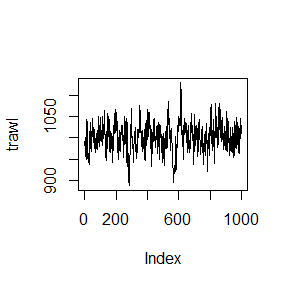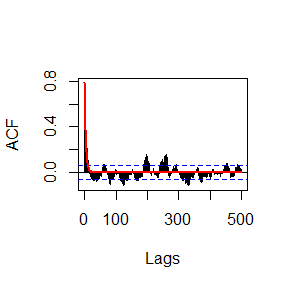#Fit the marginal Poisson law
fitmarginallaw <-trawl::fit_marginalPoisson(trawl, fittrawlfct$LM, plotdiag=TRUE)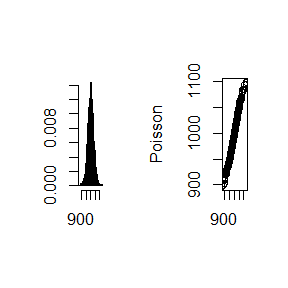###Print the results print(paste("lambda: estimated:", fittrawlfct$lambda, ", theoretical:", lambda))
#>  "lambda: estimated: 0.241963140792181 , theoretical: 0.25"
print(paste("v: estimated:", fitmarginallaw$v, ", theoretical:", v)) #>  "v: estimated: 242.197119149327 , theoretical: 250" ##Poisson with supIG trawl set.seed(1) t<-1000 Delta<-1 v<-250 delta <-0.2 gamma <-0.5 #Simulate a univariate trawl process with supIG trawl function and Poisson marginal law trawl <-trawl::sim_UnivariateTrawl(t,Delta,burnin=100,marginal =c("Poi"),trawl ="supIG",v=v, delta=delta, gamma=gamma) #Plot the sample path of the simulated process plot(trawl,type="l")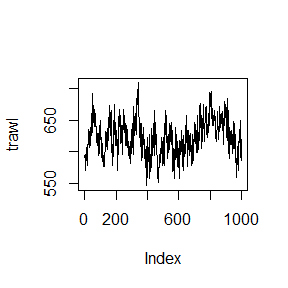#Fit the supIG trawl function to the simulated data fittrawlfct <- trawl::fit_supIGtrawl(trawl,Delta, plotacf=TRUE,lags=500)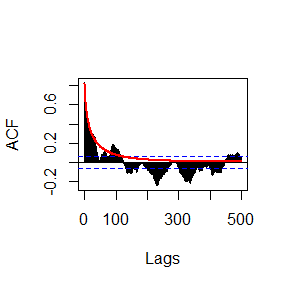#Fit the marginal Poisson law fitmarginallaw <-trawl::fit_marginalPoisson(trawl, fittrawlfct$LM, plotdiag=TRUE)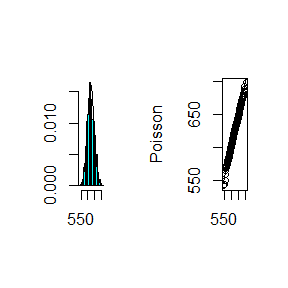###Print the results
print(paste("delta: estimated:", fittrawlfct$delta, ", theoretical:", delta)) #>  "delta: estimated: 0.189796214798363 , theoretical: 0.2" print(paste("gamma: estimated:", fittrawlfct$gamma, ", theoretical:", gamma))
#>  "gamma: estimated: 0.511685064512522 , theoretical: 0.5"
print(paste("v: estimated:", fitmarginallaw$v, ", theoretical:", v)) #>  "v: estimated: 230.833355485651 , theoretical: 250" Trawl processes with negative binomial marginal law ##Negative binomial with Exp trawl set.seed(1) t<-1000 Delta<-1 m<-200 theta<-0.5 lambda <-0.25 m*abs(log(1-theta)) #=v #>  138.6294 #Simulate a univariate trawl process with exponential trawl function and negative binomial marginal law trawl <-trawl::sim_UnivariateTrawl(t,Delta,burnin=50,marginal =c("NegBin"),trawl ="Exp",m=m, theta=theta, lambda1=lambda) #Plot the sample path of the simulated process plot(trawl,type="l")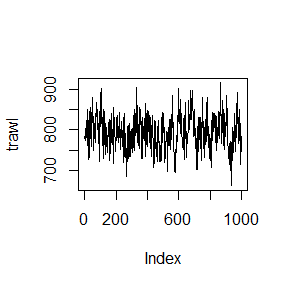#Fit the exponential trawl function to the simulated data fittrawlfct <- trawl::fit_Exptrawl(trawl,Delta, plotacf=TRUE,lags=500)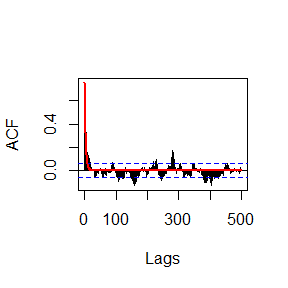#Fit the marginal negative binomial law fitmarginallaw <-trawl::fit_marginalNB(trawl, fittrawlfct$LM, plotdiag=TRUE)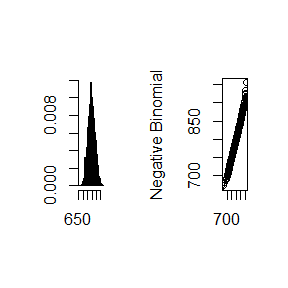###Print the results
print(paste("lambda: estimated:", fittrawlfct$lambda, ", theoretical:", lambda)) #>  "lambda: estimated: 0.281215255331676 , theoretical: 0.25" print(paste("m: estimated:", fitmarginallaw$m, ", theoretical:", m))
#>  "m: estimated: 231.550570999856 , theoretical: 200"
print(paste("theta: estimated:", fitmarginallaw$theta, ", theoretical:", theta)) #>  "theta: estimated: 0.490887447419009 , theoretical: 0.5" ##Negative binomial with LM trawl set.seed(1) t<-1000 Delta<-1 m<-200 theta<-0.5 m*abs(log(1-theta)) #=v #>  138.6294 alpha <-2 H <-3 #Simulate a univariate trawl process with long memory trawl function and negative binomial marginal law trawl <-trawl::sim_UnivariateTrawl(t,Delta,burnin=100,marginal =c("NegBin"),trawl ="LM",m=m,theta=theta, alpha=alpha, H=H) #Plot the sample path of the simulated process plot(trawl,type="l")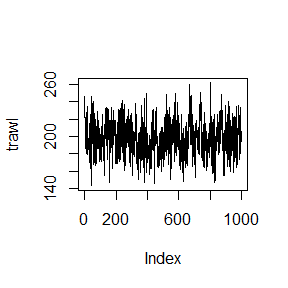#Fit the long memory trawl function to the simulated data fittrawlfct <- trawl::fit_LMtrawl(trawl,Delta, plotacf=TRUE,lags=500)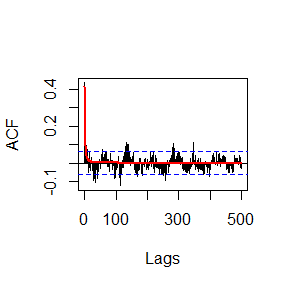#Fit the marginal negative binomial law fitmarginallaw <-trawl::fit_marginalNB(trawl, fittrawlfct$LM, plotdiag=TRUE)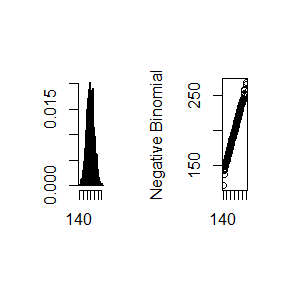###Print the results
print(paste("alpha: estimated:", fittrawlfct$alpha, ", theoretical:", alpha)) #>  "alpha: estimated: 1.87237963201705 , theoretical: 2" print(paste("H: estimated:", fittrawlfct$H, ", theoretical:", H))
#>  "H: estimated: 3.05651225671348 , theoretical: 3"
print(paste("m: estimated:", fitmarginallaw$m, ", theoretical:", m)) #>  "m: estimated: 197.98272185573 , theoretical: 200" print(paste("theta: estimated:", fitmarginallaw$theta, ", theoretical:", theta))
#>  "theta: estimated: 0.522796369302733 , theoretical: 0.5"

Simulation and inference in the bivariate case

The simulation and inference methods described above can be generalised to a multivariate setting as described in (Veraart 2017). Currently, the routines are implemented for a bivariate setting. A bivariate trawl process can be simulated using the function sim_BivariateTrawl.

In Section 3 of (Veraart 2017) two types of dependent bivariate trawl processes are discussed: the case of dependence through a common factor (see Example 8 in the underlying paper (Veraart 2017)), which is referred to as fullydep in the options of sim_BivariateTrawl, and the case of dependence through a common factor with additional independent components (see Example 9 in the underlying paper (Veraart 2017)), which is referred to as dep in the options of sim_BivariateTrawl.

The current implementation allows for simulating a bivariate trawl process with either Poisson or negative binomial marginal laws (the mixed case is possible) and the four trawl functions used in the univariate case: exponential, double-exponential, supIG and long memory.

When it comes to the inference, we provide the following functions in addition to the ones for the univariate estimation: The function fit_trawl_intersection can be used to compute the Lebesgue measure of the intersection of two trawls, where the trawl functions are either exponential, double-exponential, supIG or long-memory. More precisely, consider trawls $$A_1$$ and $$A_2$$ characterised by trawl functions $$g_1$$ and $$g_2$$. Then the function fit_trawl_intersection computes $$R_{12}(0):=\mbox{Leb}(A_1 \cap A_2)$$, where $$\mbox{Leb}$$ denotes the Lebesgue measure. For the special cases that both trawls are either exponential or long memory, the special functions fit_trawl_intersection_Exp and fit_trawl_intersection_LM are also available.

Example

We illustrate the simulation and estimation of a bivariate trawl process in the following.

#Exponential trawls
lambda1 <- 1.2
lambda2 <- 1.5

#Parameters of the negative binomial marginal law
m1<- 2.1
theta1 <- 0.9
a1 <-27.3

m2 <- 2.3
theta2 <-0.9
a2 <- 35.3

kappa12 <-m1
kappa1 <- 0
kappa2 <- m2-kappa12

#Specify the time period and grid
t <-720
Delta <- 1

##Fix the seed
set.seed(1)

#Simulate the bivariate trawl process with common factor and independent components ("dep") and
#negative binomial marginal law. Both trawl functions are chosen as exponentials.
simdata<-trawl::sim_BivariateTrawl(t, Delta, burnin=10,marginal ="NegBin", dependencetype="dep",
trawl1 ="Exp", trawl2 ="Exp",
kappa1=kappa1,kappa2=kappa2,kappa12=kappa12,a1=a1,a2=a2,
lambda11=lambda1, lambda21 =lambda2)

trawl1 <- simdata[,1]
trawl2 <- simdata[,2]

#####Produce a bivariate histogram of the simulated data
trawl::plot_2and1hist(trawl1,trawl2)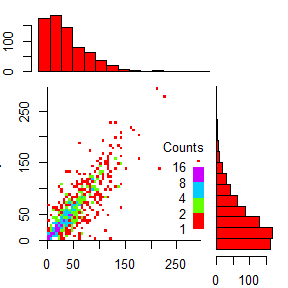###Fit the parameters of the exponential trawl functions
fit1 <- trawl::fit_Exptrawl(trawl1)
fit2 <- trawl::fit_Exptrawl(trawl2)

###Fit negative binomial model
fitNB1 <- trawl::fit_marginalNB(trawl1,fit1$LM,TRUE)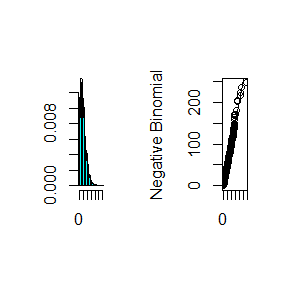fitNB2 <- trawl::fit_marginalNB(trawl2,fit2$LM,TRUE)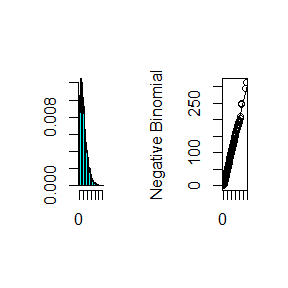###Compute the intersection between the two trawls
R12 <- trawl::fit_trawl_intersection("Exp","Exp",lambda11=fit1$memory,lambda21=fit2$memory, LM1=fit1$LM, LM2=fit2$LM,TRUE)
#>  "There are no roots"

###Fit the remaining parameters from the bivariate negative binomial law
kappa12_est <- min(stats::cov(trawl1,trawl2)/(R12*fitNB1$a*fitNB2$a),fitNB1$m,fitNB2$m)
kappa1_est <-max(fitNB1$m -kappa12,0) kappa2_est <-max(fitNB2$m -kappa12,0)

###Print the results
print("Estimated parameters of the exponential trawl functions:")
#>  "Estimated parameters of the exponential trawl functions:"
print(paste("lambda1: estimated:", fit1$lambda, ", theoretical:", lambda1)) #>  "lambda1: estimated: 1.04367205874298 , theoretical: 1.2" print(paste("lambda2: estimated:", fit2$lambda, ", theoretical:", lambda2))
#>  "lambda2: estimated: 1.37334279532044 , theoretical: 1.5"

print("Estimated parameters of the bivariate negative binomial law:")
#>  "Estimated parameters of the bivariate negative binomial law:"
print(paste("m1: estimated:", fitNB1$m, ", theoretical:", m1)) #>  "m1: estimated: 1.86651625219138 , theoretical: 2.1" print(paste("theta1: estimated:", fitNB1$theta, ", theoretical:", theta1))
#>  "theta1: estimated: 0.964798547340514 , theoretical: 0.9"
print(paste("alpha1: estimated:", fitNB1$a, ", theoretical:", a1)) #>  "alpha1: estimated: 27.4079185502173 , theoretical: 27.3" print(paste("m2: estimated:", fitNB2$m, ", theoretical:", m2))
#>  "m2: estimated: 2.22130914996701 , theoretical: 2.3"
print(paste("theta2: estimated:", fitNB2$theta, ", theoretical:", theta2)) #>  "theta2: estimated: 0.972054470109286 , theoretical: 0.9" print(paste("alpha2: estimated:", fitNB2$a, ", theoretical:", a2))
#>  "alpha2: estimated: 34.7838983161416 , theoretical: 35.3"

print(paste("kappa12: estimated:", kappa12_est, ", theoretical:", kappa12))
#>  "kappa12: estimated: 1.86651625219138 , theoretical: 2.1"
print(paste("kappa1: estimated:", kappa1_est, ", theoretical:", kappa1))
#>  "kappa1: estimated: 0 , theoretical: 0"
print(paste("kappa2: estimated:", kappa2_est, ", theoretical:", kappa2))
#>  "kappa2: estimated: 0.121309149967011 , theoretical: 0.2"